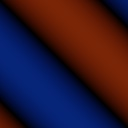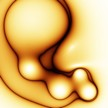## My ether wallet

Here is my ether wallet,

If you consider that some of the articles are useful, please donate:

0xEED1D1028c442de1F62969ce52D86Ba47108f561

## Genetically evolved images Part 3

In the image space presented in Part 2, we had a set of functions that measure the relative distance between two given evolved images.

Our goal now is to clusterize images, such that images that are more similar are placed into the same cluster. The usability of this feature is the efficiently split the image space according to preferences. For example one user might want to split all images that have a main motif of a circle from images that have a main motif a line.

In order to do this, we can use a clustering mechanism like k-means neighboring classification, Kohonen self-organizing maps or the DBSCAN algorithm.

These algorithms are adapted such that the distance between the images is getting smaller. Kohonen needs this in order to self-organize the image space, and k-means needs to move the centroid closer to the images. In order to do so, one can use the genetic algorithms again to create mutations such that the resulting image is a real center ( k-means) or all the images get closer to each other (Kohonen). After the genetic modifications, the "cluster" is in fact an image who is an 'average' of it's cluster, according to distances in image space.

The results indicate that the best algorithm for this purpose seem to be Kohonen, which is not dependant on initial random pick (k-means) or classification algorithms (DBSCAN).

## Genetically evolved images Part 2

Once we have defined our image representation, we can define the Image Space.

We can represent our images as trees, having a node as a function, and the operands as leaves. In this way , a more complicated image can be represented as a deeper tree, given example sin(cos(x) + y), we have the sine as root, the leaf is a plus function, which is a node having as first leaf a cosine node with a single leaf x, and the other leaf a single leaf y.

After that, we can begin to define the distance between two given images, with respect to a given distance measurement function F, in the following way:

d(i1 , i2) = F(i1 , i2)

The distance measurement function requires a list of common subtrees for the two images, which will be weightily evaluated.

We have three proposals for different distance measurement functions.

F1 = Sum (width * height * (1 / (distance to root + 1) )

F1 sums for all common subtrees, the value given by width of the subtree, its height, and 1 divided by its distance to root plus 1.

F2 = Sum (length^2 * index)

F1 sums for all common subtrees, the value given by length of subtree multiplied by its index in the common subtree list.

F3 = Sum (length * height)

We can observe that F1 is directly proportional with image complexity, as in it grows in value when the complexity grows, so comparing two simple images will result in lower values for F1, even though the images might be much more similar than ones very complex, but with a lot of common subtrees.

F2 is highly dependant on the order in which the algorithm that finds common subtrees (namely simple BF for example) indexes them.

F3 seems to be the most independent function.

Given three axes in the tridimensional Image Space, each axis representing a given value of the corresponding F function, we can place each image at a given point, starting from a reference point of a base function that we use. Further, there can be defined the Space of the Image spaces, considering that each Space can begin from a different base function. We notice that in this case simple images have a tendency to agglomerate in galaxies near the center point if they have nothing or little numbers of common subtrees with the given base function.

Note that each image is then represented as a relative distance to another, and the functions are not transitive nor having addition rules ( F1 ( i1 , i2 )  !=  F1( i1 , i3 )  +  F1( i3 , i2 ) ).

## Genetically evolved images Part 1

We can define an image representation using parametrized functions in the following way:

f(x,y) is the color of the pixel (x,y), given that very small numbers are darker colors, and very high numbers are bright colors. To obtain different colors, we can start from predefined set of 3 basic colors, and multiply them by the values give by function f. Then we can tweak the output by playing with hue and saturation. We use a clamping operator to restrict the values to [0,255] range.

This is an enhancement to the mathematical 2D representation of a function that we use in elementary school.

A few examples can contain: a constant function will represent into a uniform screen of a single color. A sinus function would then create some stripes:We can then get into more complicated images, like the following:The question at hand is how to get more images, and how to create such functions?

We will use the genetic algorithms, mainly for artificial evolution. The phenotype is represented by the resulting image itself, and the genotype is the mathematical function representation. If we are given different genotypes, we can cross-over and mutate them using genetic algorithms, in few different epochs, to obtain more complicated images. We can start from a sinus , identity, power, sum, subtraction functions, then try logarithm, etc.
This type of image generation is called evolutionary method using artificial intelligence.
All images are taken from Blot website.
Get more Joomla!® Templates and Joomla!® Forms From Crosstec